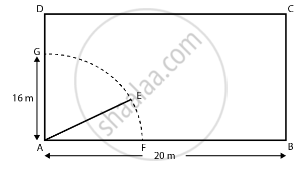# A Cow is Tied with a Rope of Length 14 M at the Corner of a Rectangular Field of Dimensions 20 M × 16 M Find the Area of the Field in Which the Cow Can Graze . - Mathematics

Sum

A cow is tied with a rope of length 14 m at the corner of a rectangular  field of dimensions 20 m xx 16 m  find the area of the field in which the cow can graze .

#### Solution

Let ABCD be a rectangular field.

Length of field = 20 m

Breadth of the field = 16 mA cow is tied at a point A.

Let length of rope be AE = 14 m = l.

Angle subtended at the center of the sector = 90°

Angle subtended at the center (in radians) θ = (90π)/180 = π/2

∴ Area of a sector of a circle = 1/2 r2θ

= 1/2 × (14)2 × (π/2)

= 154 m2

Hence, the required area of a sector of a circle is 154 m2.

Concept: Areas of Sector and Segment of a Circle
Is there an error in this question or solution?

#### APPEARS IN

NCERT Mathematics Exemplar Class 10
Chapter 11 Area Related To Circles
Exercise 11.3 | Q 5 | Page 126
RD Sharma Class 10 Maths
Chapter 13 Areas Related to Circles
Exercise 13.4 | Q 7 | Page 56
Share